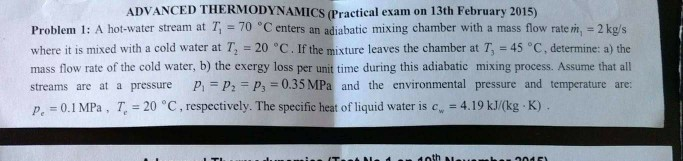# (Solved): Please Solve With Details Of Steps Used Balance Equations Flow Exergy Equation Etc Do Not Solve Usin...

please solve with details of steps used
balance equations
flow exergy equation etc
do not solve using Gouy studolas law!ADVANCED THERMODYNAMICS (Practical exam on 13th February 2015) Problem 1: A hot-water stream at Ti = 70 Â°C enters an adiabatic mixing chamber with a mass flow rate m, = 2 kg/s where it is mixed with a cold water at T, = 20 Â°C. If the mixture leaves the chamber at T = 45 Â°C, determine: a) the mass flow rate of the cold water, b) the exergy loss per unit time during this adiabatic mixing process. Assume that all streams are at a pressure P P2 = P, = 0.35 MPa and the environmental pressure and temperature are: P = 0.1 MPa, T = 20 Â°C, respectively. The specific heat of liquid water is c = 4.19 kJ/(kg-K). Moth LED

We have an Answer from Expert